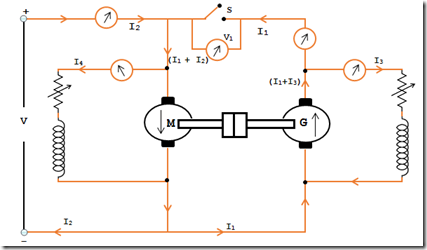# Regenerative or Hopkinson’s Test

In the previous post we have seen how to determine the efficiency of DC machines using the method Swinburn's test. Now there is an another method to determine the efficiency of a DC machines called as Regenerative method or Hopkinson’s Test method. This method saves the power and gives much accurate result of a DC machine. To carry out this test, we need similar DC machines and supply voltage.

### Principle:

Two similar DC Shunt machines are coupled mechanically and parallel connected across DC source. Both the machines; one is running as a motor and another is running as a generator by varying the shunt field excitation. The electrical power given to the motor is converted to mechanical power, the rest going to the different motor losses. This converted mechanical power given to the generator. The electrical power generated from the generator is given back to the motor except the power wasted as generator loss. Thus the electric power is taken from the DC supply is the sum of motor losses and generator losses and this can directly read through ammeter and voltmeter. Since the input power from the DC supply is equivalent to the power needed to provide the losses of two machines, this test can be done only with a small amount of power. By varying the machine field strength, we can put any load on the machine. Therefore the total loss of the machine we can determine at any load. The machines commutation performance and the temperature rise can be observed since the machine tested at full load condition.Load Sharing of DC Shunt GeneratorsThe figure above shows that typical circuit diagram of a Hopkinson’s test. Two similar DC shunt machines are coupled mechanically and parallel connected across the DC source. By varying the each machine field strength 1st machine M is allowed to run as a motor and other machine G run as a generator. The motor draws current I1 from the generator and current I2 from the DC source. Thus the current input to the motor is (I1+I2). Electrical power taken from the DC source is VI2 and which is equivalent to the total losses (motor losses and Generator losses). The shunt field current of motor is I4 and the Generator is I3.

Calculation:
Let V = Supply voltage
Motor power Input = V(I1 + I2)
Generator power Input = VI1

We can determine the efficiency of DC machines in two cases.
Case 1:  Assuming that the efficiency of both the machines are same.
Case 2:  Assuming both the machines has same iron loss, friction loss and windage loss.Characteristic of DC Shunt Generator

Case 1:
Assuming that the efficiency of both the machines are same.

Motor output power = η x Motor Input power
= η V(I1+I2)
I.e. Motor Input power = Generator Input

Now the Generator output = η x generator Input
= η x ηV(I1 +I2)
= η2 V(I1+I2)
VI1 = η2V(I1+I2)
∵ Generator output η = {I1 / (I1+I2)

Note: The above expression is used to determine the efficiency satisfactorily perfect for a rough test. If case need to find more accuracy then the efficiency of the two machines can be determined separately using the below expressions.

Case 2:
Assuming both the machines has same iron loss, friction loss and windage loss.

It is not necessary to assume that the efficiency of both the machines are same. It is due to that both the DC machines don’t have the same armature winding and the field winding. However the iron loss, friction loss and windage loss of both the machines will be same due to both the machines are identical. On this notion we can find the efficiency of each machine.

Let,
Ra = Armature winding resistance of individual machines.
I3 = Shunt field current Generator G
I4 = Shunt fief current of Motor M
Generator armature copper loss = (I1+I32) Ra
Motor armature copper loss = (I1 + I2 – I42) Ra
Shunt field copper loss in G = VI3
Shunt field copper loss in M = VI4

Power drawn from the DC source is VI2 and is equal to the total losses of motor and generator.
VI2 = Motor and Generator total losses

To get the iron loss, friction and windage loss subtract the armature copper loss and shunt copper loss of both the machine from VI2.

Total losses of 2 machines (M & G)
= VI2 – [(I1+I3)2Ra + (I1+I2-I42Ra+VI3+VI4)] = W

To find the individual machine losses divide by 2
i.e. Total losses of each machine = W/2

#### To find the efficiency of Motor

Input motor power = V(I1 + I2)
Total Losses = (I1+I2-I42)Ra + VI4 + (W/2)
= Wm

Efficiency of Motor ηm = (Input – Losses) / Input
= [V(I1+I2) – Wm] / [V(I1+I2)]

#### To find the efficiency of Generator

Generator output power = VI1
Total Losses = (W/2) + (I1+I32)Ra + VI3
= Wg

Efficiency of Generator ηg = VI1 / (VI1+Wg)This post first appeared on My Tech Info, please read the originial post: here

# Share the post

Regenerative or Hopkinson’s Test

×